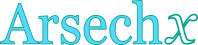## Inverse hyperbolic secant calculator

This tool evaluates the inverse hyperbolic secant of a number: arsech(x). Enter the argument x below.

 x = Result: arsech(x) =## Definitions

### General

The inverse hyperbolic secant function, in modern notation written as arsech(x) or arcsech(x) or sech-1x, gives the value t (hyperbolic angle), so that:

The inverse hyperbolic secant function accepts arguments in real interval (0,1], because for all real x. Since the hyperbolic secant is defined through the natural exponential function , its inverse can be defined through the natural logarithm function, using the following formula, for real x, with 0<x≤1:

### Properties

The derivative of the inverse hyperbolic secant function is:

The integral of the inverse hyperbolic secant function is given by: